Ratio Analysis

# Ratio Analysis - Notes | Study Cost Accounting - B Com

 1 Crore+ students have signed up on EduRev. Have you?

Problem 1:
The following is the Balance Sheet of a company as on 31st March: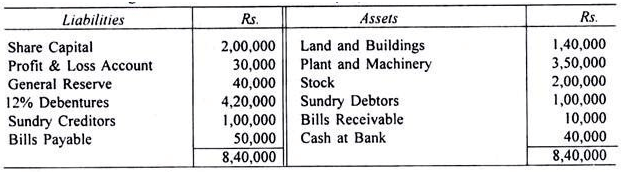Calculate :
(1) Current Ratio
(2) Quick Ratio
(3) Inventory to working Capital
(4) Debt to Equity Ratio
(5) Proprietary Ratio
(6) Capital Gearing Ratio
(7) Current Assets to Fixed Assets
Solution.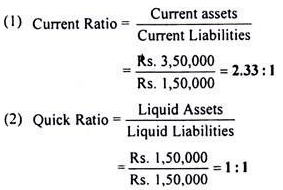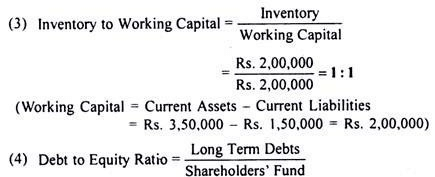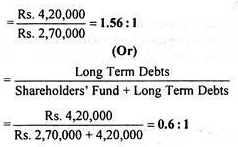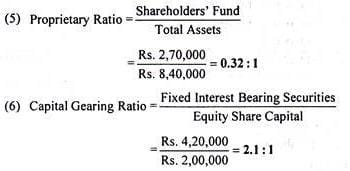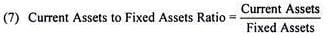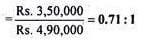Problem 2:
From the following particulars found in the Trading, Profit and Loss Account of A Company Ltd., work out the operation ratio of the business concern: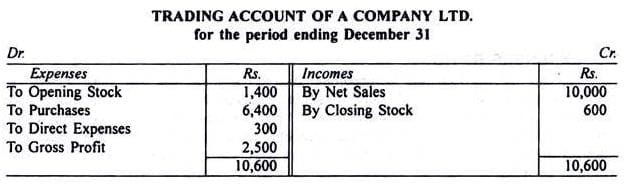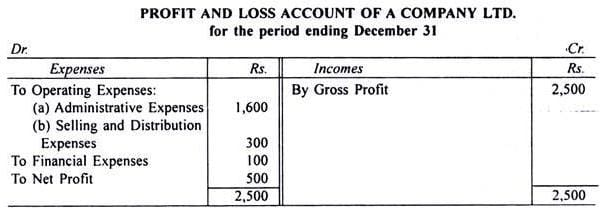Solution.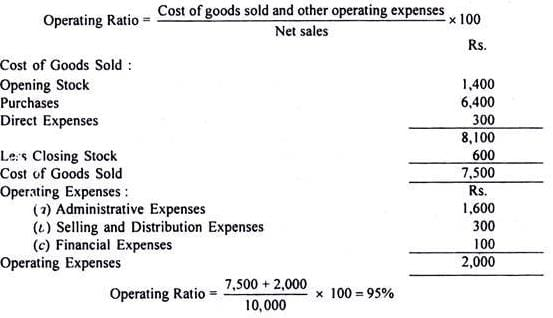Problem 3:
The following is the summarised Profit and Loss Account of Taj Products Ltd. for the year ended 31st December: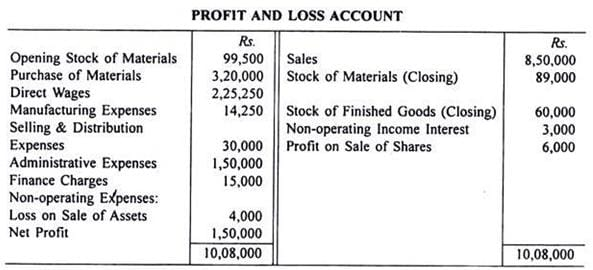Work out the following ratios:
(1) Gross Profit Ratio
(2) Net Profit Ratio
(3) Operating Ratio
(4) Cost Ratios (to cost of Production)
(i) Materials Consumed Ratio
(ii) Labour Cost Ratio
Solution.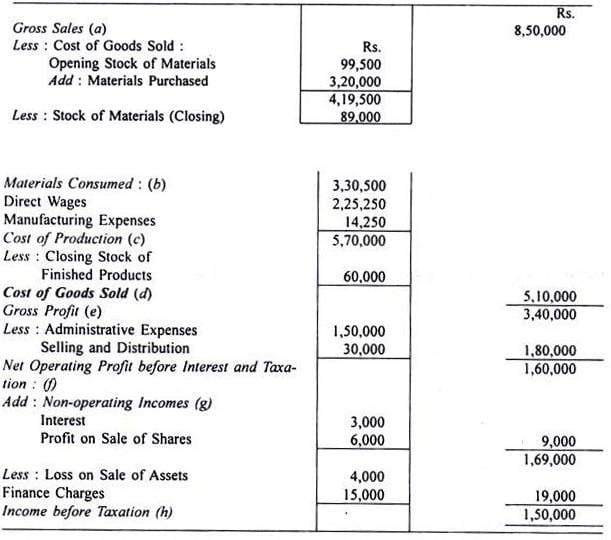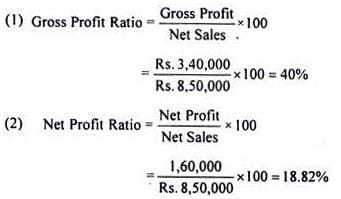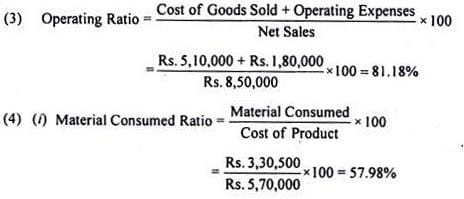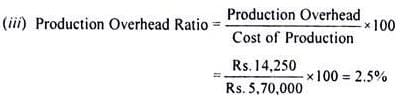Problem 4:
From the following Balance Sheet and additional information, you are required to calculate:
(i) Return on Total Resources
(ii) Return on Capital Employed
(iii) Return on Shareholders’ Fund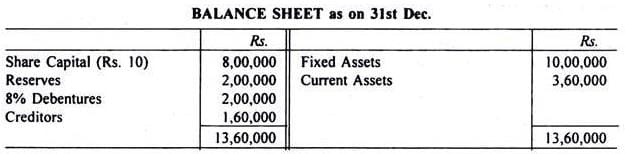Net operating profit before tax is Rs. 2,80,000. Assume tax rate at 50%. Dividend declared amounts to Rs.1,20,000.
Solution.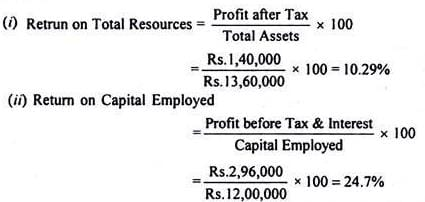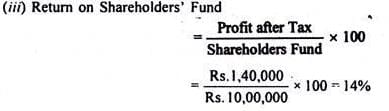Problem 5:
A company has capital of Rs. 10, 00,000; its turnover is 3 times the capital and the margin on sales is 6%. What is the return on investment.
Solution.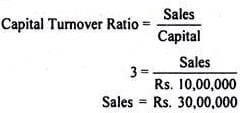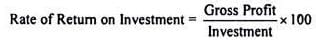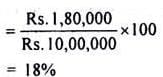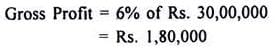Problem 6:
Ram & Company supplies you the following information regarding the year ended 31st December:
Solution.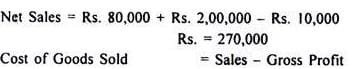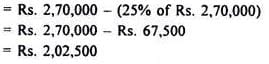Average Inventory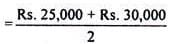Inventory Turnover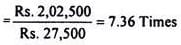Problem 7:
The following is the Profit and Loss Account of Burn Ltd.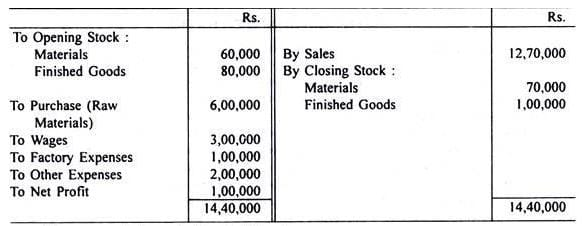You are required to calculate the following :
(i) Stock Turnover
(ii) Raw Materials Turnover
(iii) Average Material Holding
Solution.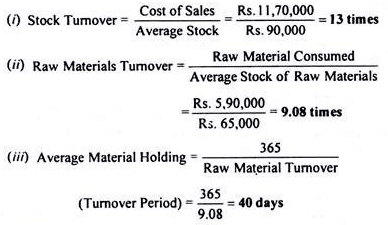Workings :
(1) Cost of Sales

= Total Cost + Opening Stock of Finished Goods - Closing Stock of Finished Goods
= Rs. 11,90,000 + Its. 80,000 - Its. 1,00,000 = Rs. 11,70,000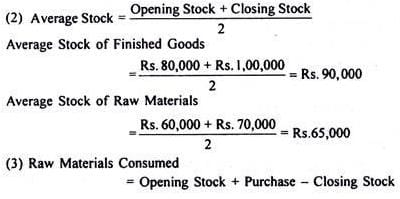= Rs. 60,000 + Rs. 6, 00,000
= Rs. 5, 90,000

Problem 8:
Calculate Debtors Velocity from the following details:
Opening Balance of Debtors Rs. 10,000
Credit Sales during the year Rs. 20,000
Sales Returns Rs. 1,000
Discount on Sales Rs. 50
Cash collected from Debtors during the year Rs, 5,000
Solution.
The following components are needed to find Debtors Velocity :
1. Net Sales = Gross Sales - (Sales Returns + Discount on Sales)
= Rs. 20,000 - (Rs. 1,000 + Rs. 50)
= Rs. 18.950
2. Net Debtors on Closing = Opening Debtors
Less : Cash Collection
Less : Sales Returns
Less : Sales Discount
Rs. 10,000 + Rs. 20,000 - (Rs. 5,000 + Rs. 1,000 + Rs. 50) = Rs. 23,950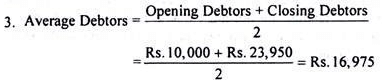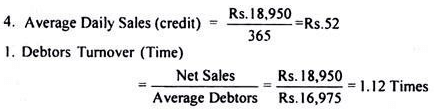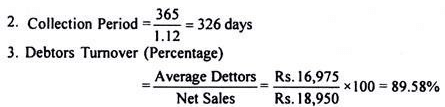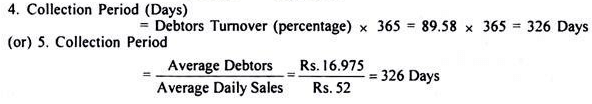Problem 9:
The Capital of a Company is as follows: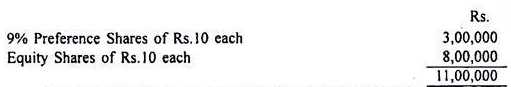The Accountant has ascertained the following information :
Profit (after tax at 60%)   Rs. 2,70,000.
Depreciation                      Rs. 60,000
Equity Dividend Paid 20%
Market Price of equity share Rs. 40.
You are required to state the following, showing the necessary workings :
(a) Dividend yield on the equity shares.
(b) Cover for the preference and equity dividends.
(c) Earnings for equity shares.
(d) Price-earnings ratio
Solution.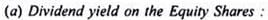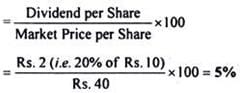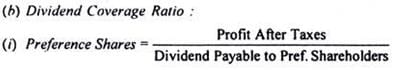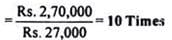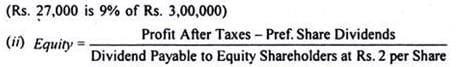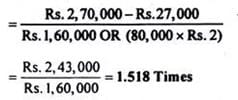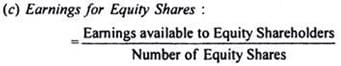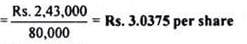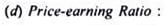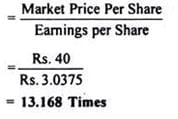Problem 10:
Assume that a firm has owners’ equity of Rs. 1, 00,000. The ratios for the firm are: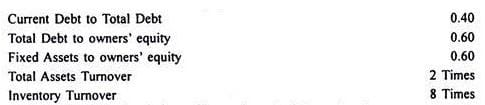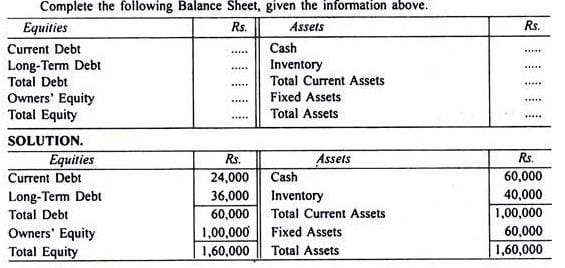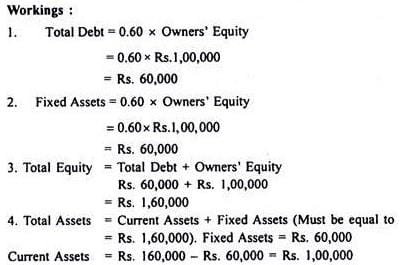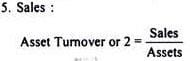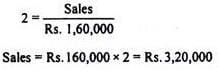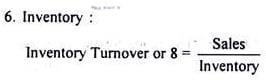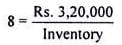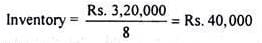7. Cash = Current Assets — Inventory
= Rs. 1,00,000 — Rs. 40,000 = Rs. 60,000
8. Current Debt = 0.40 x Total Debt = 0.40 x Rs. 60,000 = Rs. 24,000
9. Long-Term Debt = Total Debt — Current Debt = Rs. 60,000 — Rs. 24,000 = Rs. 36,000

The document Ratio Analysis - Notes | Study Cost Accounting - B Com is a part of the B Com Course Cost Accounting.
All you need of B Com at this link: B Com

## Cost Accounting

107 videos|139 docs
 Use Code STAYHOME200 and get INR 200 additional OFF

## Cost Accounting

107 videos|139 docs

Track your progress, build streaks, highlight & save important lessons and more!

,

,

,

,

,

,

,

,

,

,

,

,

,

,

,

,

,

,

,

,

,

;